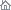Home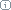About Us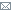ContactBlogWhat's NewProductsBuy NowDownloadsForumGeneXproTools Online Guide Learn how to use the 5 modeling platforms of GeneXproTools with the Online GuideLast update: February 19, 2014Kinds of Data

GeneXproTools supports both numerical and categorical variables, and for both types also supports missing values. Categorical and missing values are replaced automatically by simple default mappings so that you can create models straightaway, but you can choose more appropriate mappings through the Category Mapping Window and the Missing Values Mapping Window.

Numerical Data

GeneXproTools supports all kinds of tabular numerical datasets, with variables usually in columns and records in rows. In GeneXproTools all input data is converted to numerical data prior to model creation, so numerical datasets are routine for GeneXproTools and sophisticated visualization tools and statistical analyses are available in GeneXproTools for analyzing these datasets.

As long as it fits in memory, the dataset size is unlimited both for the training and validation/test datasets. However, for big datasets efficient heuristics for splitting the data are automatically applied when a run is created in order to ensure an efficient evolution and good model generalizability. Note however that these default partitioning heuristics only apply if the data is loaded as a single file; for data loaded using two different datasets you can access all the partitioning (including the default) and sub-sampling schemes of GeneXproTools in the Dataset Partitioning Window and in the General Settings Tab.

Categorical Data

GeneXproTools supports all kinds of categorical variables, both as part of entirely categorical datasets or intermixed with numerical variables. In both cases the categories in all categorical variables are automatically replaced by numerical values so that you can start modeling straightaway.

GeneXproTools uses simple heuristics to make this initial mapping, but then lets you choose more meaningful mappings in the Category Mapping Window.

Dependent categorical variables are also supported but they are handled differently in classification and logistic regression problems with more than two classes. In these cases the mapping is made so that only one class is singled out, resulting in a binomial outcome, such as {0, 1} or {-1, 1}, which can then be used to create classification or logistic regression models. The merging of the response variable in classification and logistic regression is handled in the Class Merging & Discretization Window.

In regression problems, dependent categorical variables are handled exactly as any other categorical variable, that is, the categories in the response variable are also converted to numerical values using user-defined mappings.

The beauty and power of GeneXproTools support for categorical variables goes beyond giving you access to a sophisticated and extremely useful tool for changing and experimenting with different mappings easily and quickly by trying out different scenarios and seeing immediately how they impact on modeling. Indeed GeneXproTools also generates code that supports data in exactly the same format that was loaded into GeneXproTools. This means that all the code generated both for external model deployment or for scoring internally in GeneXproTools, also supports categorical variables. Below is an example in C++ of a logistic regression model with 12 variables, 7 of which are categorical.
```
//------------------------------------------------------------------
// Logistic regression model generated by GeneXproTools 5.0 on 5/17/2013 4:13:11 PM
// GEP File: D:\GeneXproTools\Version5.0\OnlineGuide\LoanRisk_03a.gep
// Training Records:  667
// Validation Records:   333
// Fitness Function:  Bounded ROC, Logistic Threshold
// Training Fitness:  726.124343110239
// Training Accuracy: 76.76% (512)
// Validation Fitness:   777.537892479522
// Validation Accuracy:  79.58% (265)
//------------------------------------------------------------------

#include "math.h"
#include "string.h"

double gepModel(char* d_string[]);
double gep3Rt(double x);
void TransformCategoricalInputs(char* input[], double output[]);

double gepModel(char* d_string[])
{
const double G3C5 = -4.97848445081942;

double d;
TransformCategoricalInputs(d_string, d);

double dblTemp = 0.0;

dblTemp = (pow(d,4)+d);
dblTemp += exp(d);
dblTemp += (((d*pow(gep3Rt((d+G3C5)),3))-d)-d);
dblTemp += ((d*(d+d))-(((d*d)*d)*gep3Rt(d)));

const double SLOPE = 6.9596006314631E-03;
const double INTERCEPT = 3.45748287482188E-02;

double probabilityOne = 1.0 / (1.0 + exp(-(SLOPE * dblTemp + INTERCEPT)));
return probabilityOne;
}

double gep3Rt(double x)
{
return x < 0.0 ? -pow(-x,(1.0/3.0)) : pow(x,(1.0/3.0));
}

void TransformCategoricalInputs(char* input[], double output[])
{
if(strcmp("A11", input) == 0)
output = 1.0;
else if(strcmp("A12", input) == 0)
output = 2.0;
else if(strcmp("A13", input) == 0)
output = 3.0;
else if(strcmp("A14", input) == 0)
output = 4.0;
else output = 0.0;

output = atof(input);

if(strcmp("A30", input) == 0)
output = 1.0;
else if(strcmp("A31", input) == 0)
output = 2.0;
else if(strcmp("A32", input) == 0)
output = 3.0;
else if(strcmp("A33", input) == 0)
output = 4.0;
else if(strcmp("A34", input) == 0)
output = 5.0;
else output = 0.0;

if(strcmp("A61", input) == 0)
output = 1.0;
else if(strcmp("A62", input) == 0)
output = 2.0;
else if(strcmp("A63", input) == 0)
output = 3.0;
else if(strcmp("A64", input) == 0)
output = 4.0;
else if(strcmp("A65", input) == 0)
output = 5.0;
else output = 0.0;

output = atof(input);

if(strcmp("A91", input) == 0)
output = 1.0;
else if(strcmp("A92", input) == 0)
output = 2.0;
else if(strcmp("A93", input) == 0)
output = 3.0;
else if(strcmp("A94", input) == 0)
output = 4.0;
else output = 0.0;

if(strcmp("A101", input) == 0)
output = 1.0;
else if(strcmp("A102", input) == 0)
output = 2.0;
else if(strcmp("A103", input) == 0)
output = 3.0;
else output = 0.0;

output = atof(input);

if(strcmp("A121", input) == 0)
output = 1.0;
else if(strcmp("A122", input) == 0)
output = 2.0;
else if(strcmp("A123", input) == 0)
output = 3.0;
else if(strcmp("A124", input) == 0)
output = 4.0;
else output = 0.0;

output = atof(input);

output = atof(input);

if(strcmp("A191", input) == 0)
output = 1.0;
else if(strcmp("A192", input) == 0)
output = 2.0;
else output = 0.0;
}
```

Missing Values

GeneXproTools supports missing values both for numerical and categorical variables. The supported representations for missing values consist of NULL, Null, null, NA, na, ?, blank cells, ., ._, and .*, where * can be any letter in lower or upper case.

When data is loaded into GeneXproTools, the missing values are automatically replaced by zero so that you can start modeling right away. But then GeneXproTools allows you to choose different mappings through the Missing Values Mapping Window.

In the Missing Values Mapping Window you have access to pre-computed data statistics, such as the majority class for categorical variables and the average for numerical variables, to help you choose the most effective mapping.

As mentioned above for categorical values, GeneXproTools is not just a useful platform for trying out different mappings for missing values to see how they impact on model evolution and then choose the best one: GeneXproTools generates code with support for missing values that you can immediately deploy without further hassle, allowing you to use the exact same format that was used to load the data into GeneXproTools. The sample MATLAB code below shows a classification model of 7 variables, 6 of which with missing values:
```
%------------------------------------------------------------------
% Classification model generated by GeneXproTools 5.0 on 5/17/2013 6:44:02 PM
% GEP File: D:\GeneXproTools\Version5.0\OnlineGuide\Diabetes_M01.gep
% Training Records:  570
% Validation Records:   198
% Fitness Function:  ROC Measure, ROC Threshold
% Training Fitness:  801.044268510405
% Training Accuracy: 75.09% (428)
% Validation Fitness:   842.459561470235
% Validation Accuracy:  77.27% (153)
%------------------------------------------------------------------

function result = gepModel(d_string)

ROUNDING_THRESHOLD = 1444302.57350085;

G1C9 = 8.49354625080111;
G2C0 = -3.496505630665;
G2C6 = 0.893559068575091;
G3C6 = 4.40351573229164;

d = TransformCategoricalInputs(d_string);

varTemp = 0.0;

varTemp = ((gep3Rt(((d(4)-d(1))^3))-(d(8)*(d(6)-G1C9)))^2);
varTemp = varTemp + (((((G2C0+d(2))/2.0)*(d(2)+d(2)))+((G2C6+d(5))*G2C0))*d(2));
varTemp = varTemp + (gep3Rt((d(2)*(((G3C6-d(2))*d(3))-d(5))))^3);

if (varTemp >= ROUNDING_THRESHOLD),
result = 1;
else
result = 0;
end

function result = gep3Rt(x)
if (x < 0.0),
result = -((-x)^(1.0/3.0));
else
result = x^(1.0/3.0);
end

function output = TransformCategoricalInputs(input)
switch char(input(1))
case '.D'
output(1) = 12.0;
case '.E'
output(1) = 11.0;
case '.L'
output(1) = 15.0;
case '.T'
output(1) = 10.0;
case '.Z'
output(1) = 0.0;
otherwise
output(1) = str2double(input(1));
end

switch char(input(2))
case '?'
output(2) = 0.0;
otherwise
output(2) = str2double(input(2));
end

switch char(input(3))
case '?'
output(3) = 0.0;
otherwise
output(3) = str2double(input(3));
end

switch char(input(4))
case '?'
output(4) = 0.0;
otherwise
output(4) = str2double(input(4));
end

switch char(input(5))
case '?'
output(5) = 0.0;
otherwise
output(5) = str2double(input(5));
end

switch char(input(6))
case '?'
output(6) = 0.0;
otherwise
output(6) = str2double(input(6));
end

output(8) = str2double(input(8));
```Datasets

Through the Dataset Partitioning Window and the sub-sampling schemes in the General Settings Tab, GeneXproTools allows you to split your data into different datasets that can be used to:

• Create the models (the training dataset or a sub-set of the training dataset).
• Check and select the models during the design process (the validation dataset or a sub-set of the validation dataset).
• Test the final model (a sub-set of the validation set reserved for testing).
Of all these datasets, the training dataset is the only one that is mandatory as GeneXproTools requires data to create data models. The validation and test sets are optional and you can indeed create models without checking or testing them. Note however that this approach is not recommended and you have indeed better chances of creating good models if you check their generalizability regularly not only during model design but also during model selection. However if you don’t have enough data, you can still create good models with GeneXproTools as the learning algorithms of GeneXproTools are not prone to overfitting the data. In addition, if you are using GeneXproTools to create random forests, the need for validating/testing the models of the ensemble is less important as ensembles tend to generalize better than individual models.

Training Dataset

The training dataset is used to create the models, either in its entirety or as a sub-sample of the training data. The sub-samples of the training data are managed in the Settings Panel.

GeneXproTools supports different sub-sampling schemes, such as bagging and mini-batch. For example, to operate in bagging mode you just have to set the sub-sampling to Random. In addition, you can also change the number of records used in each bag, allowing you to speed up evolution if you have enough data to get good generalization.

Besides Random Sampling (which is done with replacement) you can also choose Shuffled (which is done without replacement), Balanced Random (used in classification and logistic regression runs where a sub-sample is randomly generated so that the proportion of positives and negatives are the same), and Balanced Shuffled (similar to Balanced Random, but with the sampling done without replacement).

All types of random sampling can be used in mini-batch mode, which is an extremely useful sampling method for handling big datasets. In mini-batch mode a sub-sampling of the training data is generated each p generations (the period of the mini-batch, which is adjustable and can be set in the Settings Panel) and is used for training during that period. This way, for large datasets good models can be generated quickly using an overall high percentage of the records in the training data, without stalling the whole evolutionary process with a huge dataset that is used each generation. It’s important however to find a good balance between the size of the mini-batch and an efficient model evolution. This means that you’ll still have to choose an appropriate number of records in order to ensure good generalizability, which is true for all datasets, big and small. A simple rule of thumb is to see if the best fitness is increasing overall: if you see it fluctuating up and down, evolution has stalled and you need either to increase the mini-batch size or increase the time between batches (the period). The chart below shows clearly the overall upward trend in best fitness for a run in mini-batch mode with a period of 50.

The training dataset is also used for evaluating data statistics that are used in certain models, such as the average and the standard deviation of predictor variables used in models created with standardized data. Other data statistics used in GeneXproTools include: pre-computed suggested mappings for missing values; training data constants used in Excel worksheets both for models and ensembles deployed to Excel; min and max values of variables when normalized data is used (0/1 Normalization and Min/Max Normalization), and so on.

It’s important to note that when a sub-set of the training dataset is used in a run, the training data constants pertain to the data constants of the entire training dataset as defined in the Data Panel. For example, this is important when designing ensemble models using different random sampling schemes selected in the Settings Panel.

On the other hand when a sub-set of the training dataset is used to evolve a model, the model statistics or constants, if they exist, remain fixed and become an integral part of the evolved model. Examples of such model parameters include the evolvable rounding thresholds of classification models and logistic regression models.

In addition to random sampling schemes, GeneXproTools supports non-random sampling schemes, such as using just the odd or even records; the top half or bottom half records; and the top n or bottom n records.

Validation Dataset

GeneXproTools supports the use of a validation dataset, which can be either loaded as a separate dataset or generated from a single dataset using GeneXproTools partitioning algorithms. Indeed if during the creation of a new run a single dataset is loaded, GeneXproTools automatically splits the data into Training and Validation/Test datasets. GeneXproTools uses optimal strategies to split the data in order to ensure good model design and evolution. These default partition strategies offer useful guidelines, but you can choose different partitions in the Dataset Partitioning Window to meet your needs. For instance, the Odds/Evens partition is useful for times series data, allowing for a good split without losing the time dimension of the original data, which obviously can help in better understanding both the data and the generated models.

GeneXproTools also supports sub-sampling for the validation dataset, which, as explained for the training dataset above, is controlled in the Settings Panel.

The sub-sampling schemes available for the validation data are exactly the same available for the training data, except of course for the mini-batch strategy which pertains only to the training data.

It’s worth pointing out, however, that the same sampling scheme in the training and validation data can play very different roles. For example, by choosing the Odds or the Evens, or the Bottom Half or Top Half for validation, you can reserve the other part for testing and only use this test dataset at the very end of the modeling process to evaluate the accuracy of your model.

Another ingenious use of all the random sampling schemes available for the validation set (Random, Shuffled, Balanced Random and Balanced Shuffled, with the last two available only for Classification, Logistic Regression and Logic Synthesis) consists of calculating the cross-validation accuracy of a model. A clever and simple way to do this, consists of creating a run with the model you want to cross-validate. Then you can copy this model n times, for instance by importing it n times. Then in the History Panel you can evaluate the performance of the model for different sub-samples of the validation dataset. The average value for the fitness and favorite statistic shown in the statistics summary of the History Panel consist of the cross-validation results for your model. Below is an example of a 30-fold cross-validation evaluated for the training and validation datasets using random sampling of the respective datasets.

Test Dataset

The dividing line between a test dataset and a validation dataset is not always clear. A popular definition comes from modeling competitions, where part of the data is hold out and not accessible to the people doing the modeling. In this case, of course, there’s no other choice: you create your model and then others check if it is any good or not. But in most real situations people do have access to all the data and they are the ones who decide what goes into training, validation and testing.

GeneXproTools allows you to experiment with all these scenarios and you can choose what works best for the data and problem you are modeling. So if you want to be strict, you can hold out part of the data for testing and load it only at the very end of the modeling process, using the Change Validation Dataset functionality of GeneXproTools.

Another option is to use the technique described above for the validation dataset, where you hold out part of the validation data for testing. For example, you hold out the Odds or the Evens, or the Top Half or Bottom Half. This obviously requires strong willed and very disciplined people, so it’s perhaps best practiced only if a single person is doing the modeling.

The takeaway message of all these what-if scenarios is that, after working with GeneXproTools for a while, you’ll be comfortable with what is good practice in testing the accuracy of the models you create with GeneXproTools. We like to claim that the learning algorithms of GeneXproTools are not prone to overfitting and now with all the partitioning and sampling schemes of GeneXproTools you can develop a better sense of the quality of the models generated by GeneXproTools.

And finally, the same cross-validation technique described above for the validation dataset can be performed for the test dataset.Loading Data

Before evolving a model with GeneXproTools you must first load the input data for the learning algorithms. GeneXproTools allows you to work with text files, Excel/databases and GeneXproTools files.

Text Files

For text files GeneXproTools supports three different data formats.

The first is the standard Records x Variables format where records are in rows and variables in columns, with the dependent or response variable occupying the rightmost position.

In the small example below with 10 records, IRIS_PLANT is the response variable and SEPAL_LENGTH, SEPAL_WIDTH, PETAL_LENGTH, and PETAL_WIDTH are the independent or predictor variables:

SEPAL_LENGTH SEPAL_WIDTH PETAL_LENGTH PETAL_WIDTH IRIS_PLANT
5.4 3.4 1.7 0.2 0
6.1 3.0 4.6 1.4 0
5.0 3.4 1.6 0.4 0
5.2 3.5 1.5 0.2 0
5.1 3.7 1.5 0.4 0
5.5 2.4 3.7 1.0 0
7.2 3.2 6.0 1.8 1
6.3 2.7 4.9 1.8 1
7.7 3.8 6.7 2.2 1
4.8 3.4 1.9 0.2 0

The second format is similar to the first, but the response variable is in the first column.

And the third is the Gene Expression Matrix format commonly used in DNA microarrays studies where records are in columns and variables in rows, with the response occupying the topmost position. For instance, in Gene Expression Matrix format, the small dataset above corresponds to:

IRIS_PLANT 0 0 0 0 0 0 1 1 1 0
SEPAL_LENGTH 5.4 6.1 5.0 5.2 5.1 5.5 7.2 6.3 7.7 4.8
SEPAL_WIDTH 3.4 3.0 3.4 3.5 3.7 2.4 3.2 2.7 3.8 3.4
PETAL_LENGTH 1.7 4.6 1.6 1.5 1.5 3.7 6.0 4.9 6.7 1.9
PETAL_WIDTH 0.2 1.4 0.4 0.2 0.4 1.0 1.8 1.8 2.2 0.2

which is very useful for datasets with a relatively small number of records and thousands of variables. Note, however, that for Excel files this format is not supported and if your data is kept in this format in Excel, you must copy it to a text file so that it can be loaded into GeneXproTools.

GeneXproTools uses the Records x Variables format with the response at the end internally and therefore all input formats are automatically converted and shown in this format.

GeneXproTools supports the standard separators (space, tab, comma, semicolon, and pipe) and detects them automatically. The use of labels to identify your variables is optional and GeneXproTools also detects automatically whether they are present or not. If you use them, however, you will be able to generate more intelligible code where each variable is identified by its name, by checking the Use Labels box in the Model Panel.

Excel Files & Databases

The loading of data from Excel/databases requires making a connection with Excel/database and then selecting the worksheets or columns of interest.

GeneXproTools Files

GeneXproTools files can be very convenient as they allow the selection of exactly the same datasets used in a run. This can be very useful especially if you want to use the same datasets across different problem categories or across different runs.Problem Categories

The new algorithms for loading data into GeneXproTools, with their support for categorical variables, impose almost no constraints in the datasets required for a particular problem category.

Regression

Function Finding or Regression problems require a numeric dependent variable to create regression models. Now with the support for categorical variables, which was also extended to the dependent variable, datasets with categorical dependent variables can now be loaded into GeneXproTools too and used in regression problems, as GeneXproTools allows the mapping of all categorical values to numbers. For example, a dependent variable with nominal ratings such as {Excellent, Good, Satisfactory, Bad} can be easily used in regression with the mapping {4, 3, 2, 1}.

Classification & Logistic Regression

For Classification and Logistic Regression the learning algorithms of GeneXproTools require a binomial response variable. Here, combining the support for categorical variables with the merging and discretization tools of GeneXproTools, datasets with multiple classes and datasets with numerical responses (both continuous and discrete) can be used for creating classification and logistic regression models.

For datasets with multiple classes GeneXproTools allows you to set the singled out class to C1 for example, and then create models for the binomial classification task {class C1, not class C1}. Then single out another class and create models for it too, and so on until you've created models for all sub-tasks.

On the other hand, for datasets with a numerical response variable (loosely defined as having more than two different values), such as the output of a logistic regression model with continuous probability values between [0, 1], you can use the discretization function of GeneXproTools and easily convert the continuous output into a binomial outcome of {0, 1} by choosing 0.5 as the discretization threshold.

Time Series Prediction

Time series prediction models require a time series loaded as a single column into GeneXproTools.

For text files, only files with a single column of observations can be used for loading the time series into GeneXproTools.

For Excel files and databases, the loading is more flexible as GeneXproTools allows the use of Excel files with multiple columns, from which you can select the column of interest to load into GeneXproTools.

After loading the time series, GeneXproTools transforms the time series so that it can be used to create dynamic regression models with GeneXproTools learning algorithms. Some initial values for the embedding dimension, the delay time and the prediction mode are required for transforming the time series during the creation of a new run, but you can change these parameters also in the Settings Panel.

Time series data with missing values are supported, and GeneXproTools replaces all missing values automatically by zero. Note however that this mapping cannot be changed afterwards.

Logic Synthesis

Logic synthesis models are created using Boolean inputs, such as {0, 1} or {true, false}. GeneXproTools supports both representations, although internally in all the charts and tables GeneXproTools shows only 0’s and 1’s.

And finally, missing values are not supported in logic synthesis files.

Related Tutorials:

Related Videos:

 Leave Feedback Please enter the number below using the combo boxes before sending your feedback. 3 8 4 0 1 2 3 4 5 6 7 8 9   0 1 2 3 4 5 6 7 8 9   0 1 2 3 4 5 6 7 8 9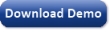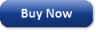Time Limited Trial

Released February 19, 2014

Last update: 5.0.3883New Entries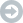Subscribe to the GEP-List

 3 8 4 0 1 2 3 4 5 6 7 8 9   0 1 2 3 4 5 6 7 8 9   0 1 2 3 4 5 6 7 8 9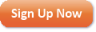Home | What's New | Products | Buy Now | Downloads | Quick Tour | Support | Contact Us | About Gepsoft | Sign UpForum | Blog | Videos | Tutorials | Server Knowledge Base | Logistic Regression Guide | Terms of Use | Privacy & Cookies